# LDraw.org Discussion Forums

You're currently viewing a stripped down version of our content. View the full version with proper formatting.
Pages: 1 2 3 4 5 6 7 8 9 10 11
The implementation of the BFC specification (what is Backface Culling?) into LDPartEditor is done.

All BFC changes will be applied instantly.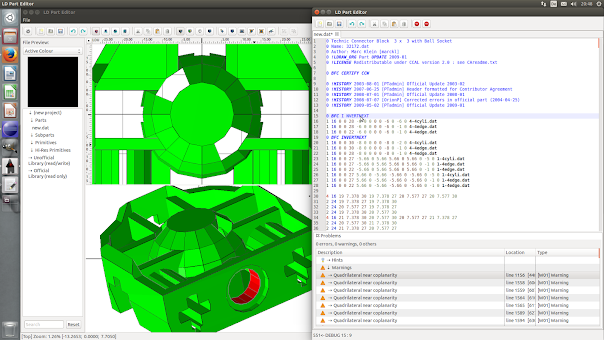A "BFC NOCERTIFY" among others: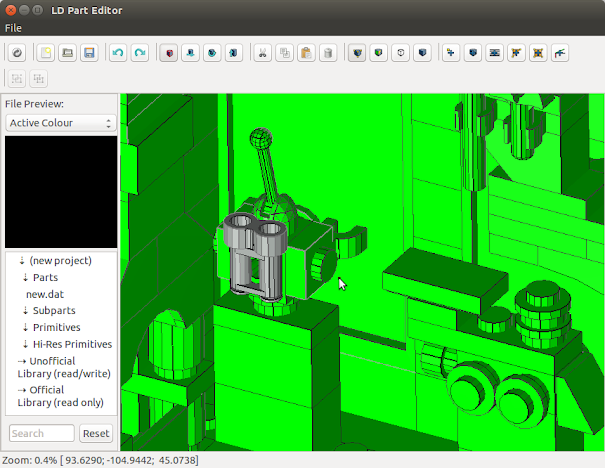BFC CLIP / NOCLIP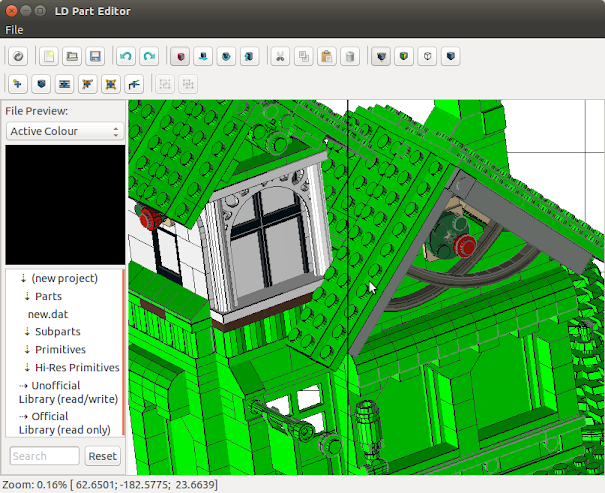Here is another example of multiple views. The screenshot was taken before I finished the BFC feature.
Closer and closer to release...???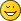Even a pre-alpha would do it. Better soon than perfect.

w.
I fully agree with WillyThe next implementation step were optional lines.

There are different ways to display these lines, e.g.:

1. Show all
2. Show all + control points
3. Draw them as described in the official specification
4. " + control points

However.. I will improve the rendering of (optional) lines in the future.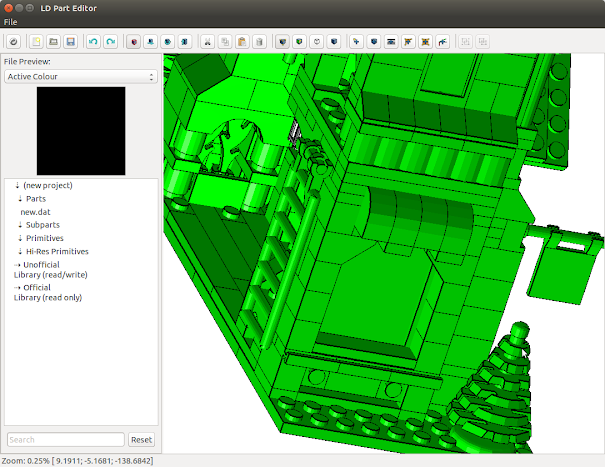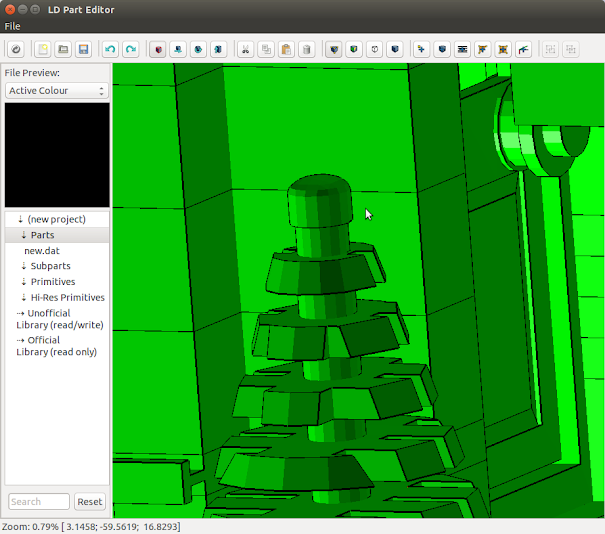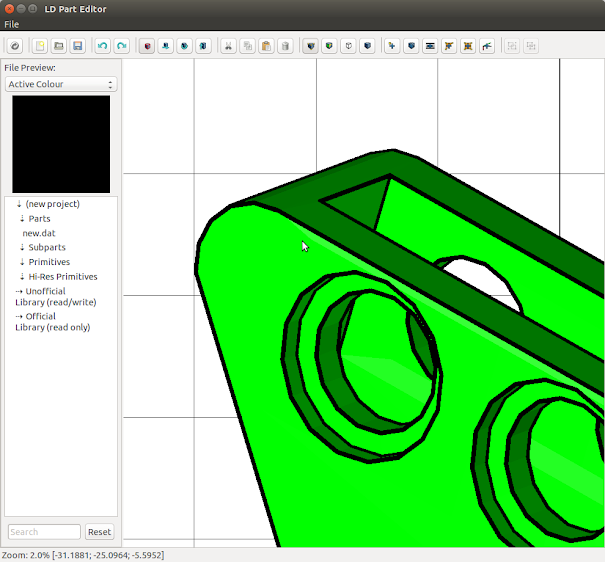edit: Two new pictures from the vertex development processHow do you go about rendering conditional lines like that..? It apparently is a big hassle when you do it with OpenGL. What's the stunt here?
Imagine an optional line with the four points A2, B2, C2 and D2.

1. Take these points and multiply them by their perspective and modelview matrix M.
As a result you'll get A, B, C and D in screen coordinates.

Code:
```Matrix4f.transform(M, A2, A); Matrix4f.transform(M, B2, B); Matrix4f.transform(M, C2, C); Matrix4f.transform(M, D2, D);```

2. Calculate the line normal

Code:
```N.x = A.y - B.y; N.y = B.x - A.x;```

3. Calculate the discriminant r by

Code:
`[b]r[/b] = Vector4f.dot(N, Vector4f.sub(C, A)) * Vector4f.dot(N, Vector4f.sub(D, A));`

where Vector4f.dot() calculates the dot product of these two vectors and Vector4f.sub() the difference vector.

4. draw the line between A2 and B2 only if r > 0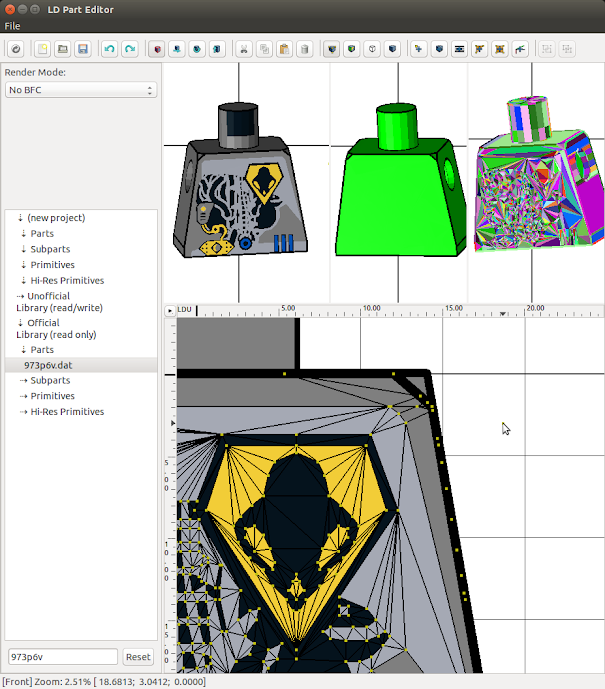Big question is: Will I be able to select a vertex, move it with the mouse in 3D and all lines, cond. lines, triangles, quads connected will be affected by the move?

w.
Pages: 1 2 3 4 5 6 7 8 9 10 11